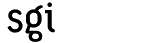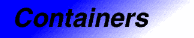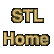# hash_multiset<Key, HashFcn, EqualKey, Alloc>Category: containers Component type: type

### Description

Hash_multiset is a Hashed Associative Container that stores objects of type Key. Hash_multiset is a simple associative container, meaning that its value type, as well as its key type, is Key. It is also a Multiple Associative Container, meaning that two or more elements may compare equal using the Binary Predicate EqualKey.

Hash_multiset is useful in applications where it is important to be able to search for an element quickly. If it is important for the elements to be in a particular order, however, then multiset is more appropriate.

### Example

```struct eqstr
{
bool operator()(const char* s1, const char* s2) const
{
return strcmp(s1, s2) == 0;
}
};

void lookup(const hash_multiset<const char*, hash<const char*>, eqstr>& Set,
const char* word)
{
int n_found = Set.count(word);
cout << word << ": "
<< n_found << " "
<< (n_found == 1 ? "instance" : "instances")
<< endl;
}

int main()
{
hash_multiset<const char*, hash<const char*>, eqstr> Set;
Set.insert("mango");
Set.insert("kiwi");
Set.insert("apple");
Set.insert("kiwi");
Set.insert("mango");
Set.insert("mango");
Set.insert("apricot");
Set.insert("banana");
Set.insert("mango");

lookup(Set, "mango");
lookup(Set, "apple");
lookup(Set, "durian");
}
```

### Definition

Defined in the header hash_set, and in the backward-compatibility header hash_set.h. This class is an SGI extension; it is not part of the C++ standard.

### Template parameters

Parameter Description Default
Key The hash_multiset's key type and value type. This is also defined as hash_multiset::key_type and hash_multiset::value_type
HashFcn The Hash Function used by the hash_multiset. This is also defined as hash_multiset::hasher. hash<Key>
EqualKey The hash_multiset's key equality function: a binary predicate that determines whether two keys are equal. This is also defined as hash_multiset::key_equal. equal_to<Key>
Alloc The hash_multiset's allocator, used for all internal memory management. alloc

### Model of

Multiple Hashed Associative Container, Simple Associative Container

None.

### Members

Member Where defined Description
value_type Container The type of object, T, stored in the hash_multiset.
key_type Associative Container The key type associated with value_type.
hasher Hashed Associative Container The hash_multiset's Hash Function.
key_equal Hashed Associative Container Function object that compares keys for equality.
pointer Container Pointer to T.
reference Container Reference to T
const_reference Container Const reference to T
size_type Container An unsigned integral type.
difference_type Container A signed integral type.
iterator Container Iterator used to iterate through a hash_multiset.
const_iterator Container Const iterator used to iterate through a hash_multiset. (Iterator and const_iterator are the same type.)
iterator begin() const Container Returns an iterator pointing to the beginning of the hash_multiset.
iterator end() const Container Returns an iterator pointing to the end of the hash_multiset.
size_type size() const Container Returns the size of the hash_multiset.
size_type max_size() const Container Returns the largest possible size of the hash_multiset.
bool empty() const Container true if the hash_multiset's size is 0.
size_type bucket_count() const Hashed Associative Container Returns the number of buckets used by the hash_multiset.
void resize(size_type n) Hashed Associative Container Increases the bucket count to at least n.
hasher hash_funct() const Hashed Associative Container Returns the hasher object used by the hash_multiset.
key_equal key_eq() const Hashed Associative Container Returns the key_equal object used by the hash_multiset.
hash_multiset() Container Creates an empty hash_multiset.
hash_multiset(size_type n) Hashed Associative Container Creates an empty hash_multiset with at least n buckets.
```hash_multiset(size_type n,
const hasher& h)
```
Hashed Associative Container Creates an empty hash_multiset with at least n buckets, using h as the hash function.
```hash_multiset(size_type n,
const hasher& h,
const key_equal& k)
```
Hashed Associative Container Creates an empty hash_multiset with at least n buckets, using h as the hash function and k as the key equal function.
```template <class InputIterator>
hash_multiset(InputIterator, InputIterator)

```
Multiple Hashed Associative Container Creates a hash_multiset with a copy of a range.
```template <class InputIterator>
hash_multiset(InputIterator, InputIterator,
size_type n)

```
Multiple Hashed Associative Container Creates a hash_multiset with a copy of a range and a bucket count of at least n.
```template <class InputIterator>
hash_multiset(InputIterator, InputIterator,
size_type n, const hasher& h)

```
Multiple Hashed Associative Container Creates a hash_multiset with a copy of a range and a bucket count of at least n, using h as the hash function.
```template <class InputIterator>
hash_multiset(InputIterator, InputIterator,
size_type n, const hasher& h,
const key_equal& k)

```
Multiple Hashed Associative Container Creates a hash_multiset with a copy of a range and a bucket count of at least n, using h as the hash function and k as the key equal function.
hash_multiset(const hash_multiset&) Container The copy constructor.
hash_multiset& operator=(const hash_multiset&) Container The assignment operator
void swap(hash_multiset&) Container Swaps the contents of two hash_multisets.
iterator insert(const value_type& x) Multiple Associative Container Inserts x into the hash_multiset.
```template <class InputIterator>
void insert(InputIterator, InputIterator)

```
Multiple Associative Container Inserts a range into the hash_multiset.
void erase(iterator pos) Associative Container Erases the element pointed to by pos.
size_type erase(const key_type& k) Associative Container Erases the element whose key is k.
void erase(iterator first, iterator last) Associative Container Erases all elements in a range.
void clear() Associative Container Erases all of the elements.
iterator find(const key_type& k) const Associative Container Finds an element whose key is k.
size_type count(const key_type& k) const Associative Container Counts the number of elements whose key is k.
```pair<iterator, iterator>
equal_range(const key_type& k) const
```
Associative Container Finds a range containing all elements whose key is k.
```bool operator==(const hash_multiset&,
const hash_multiset&)
```
Hashed Associative Container Tests two hash_multisets for equality. This is a global function, not a member function.

### New members

All of hash_multiset's members are defined in the Multiple Hashed Associative Container and Simple Associative Container requirements. Hash_multiset does not introduce any new members.

### Notes

 This member function relies on member template functions, which at present (early 1998) are not supported by all compilers. If your compiler supports member templates, you can call this function with any type of input iterator. If your compiler does not yet support member templates, though, then the arguments must either be of type const value_type* or of type hash_multiset::const_iterator.